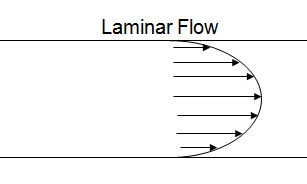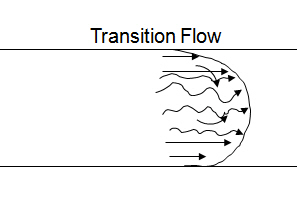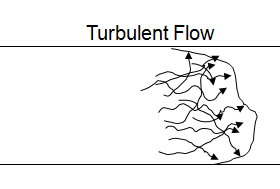# Flow Regime and Critical Reynolds Number for Drilling Hydraulics

Drilling fluid has three flow regimes which are laminar, transition or turbulent flow. The following illustrations demonstrate three types of flowIn 1883 when Mr Osborne Reynolds did the fluid study, he discovered Reynolds number describing flow of water in a circular pipe. From his experiment, the turbulent flow starts at the Reynolds Number of 2,000 and the complete turbulent flow occurs at the Reynolds Number of 4,000. Therefore, when the calculated Reynolds number of fluid is between 2,000 and 4,000, the transition flow is called.

The Reynolds Number describing flow changes is not the same as water because behavior of drilling mud does not act exactly like water. In order to get the accurate flow regime for drilling fluid, the following equations are developed to determine the critical Reynolds Number.

Laminar flow:

Rec = 3,470 – 1,370na

Transition flow:

3,470 – 1,370na < Rec < 4,270 – 1,370na

Turbulent flow:

Rec = 4,270 – 1,370na

Where;

Rec = critical Reynolds number in an annular

na = power law constant of drilling fluids

Reference:Drilling Hydraulic Books

Share the joy